##Hyperbola property

Given two intersecting lines e = AB, f = AC and a point D not on these lines, consider all lines through D and their intersection points G, H with e, f. Take the symmetric point I of A with respect to the middle of GH. The geometric locus of I is a hyperbola with asymptotic lines parallel to e, f, passing through D. Its equation with respect to the system of its asymptotics is x*y = a*b, with a = |DJ| and b = |DE|.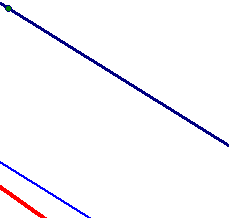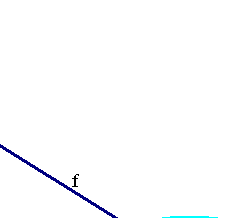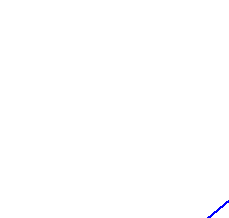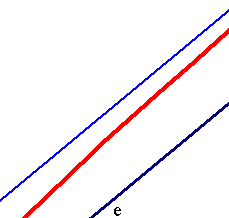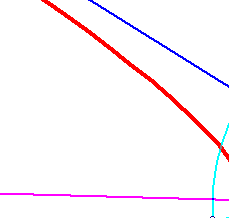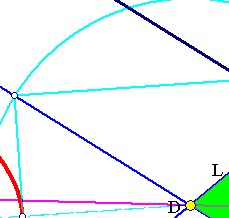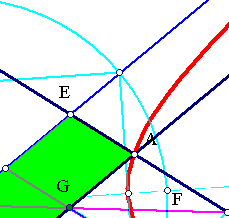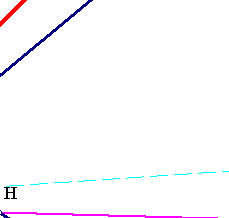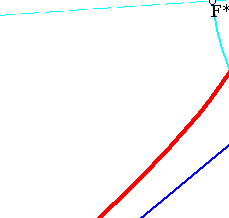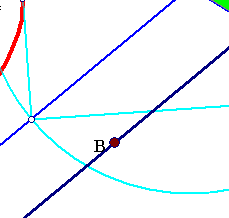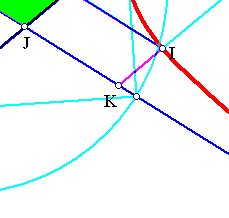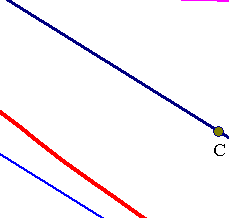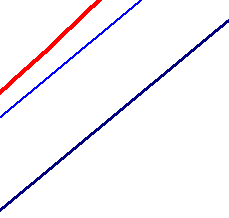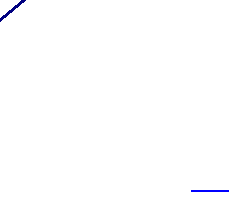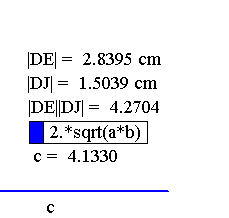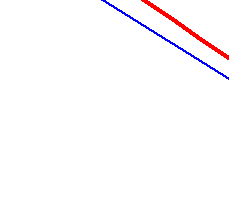In fact, from the similar parallelogramms DEHK and GAHI one has x/b = (x-a)/(b-y), x = DK and y = IK being the coordinates of I with respect to the lines DJ, DE, parallel to AC and AB respectively. It follows that x*y = a*b. The calculation above defines c through a*b = c˛/4. The rest and the construction procedure results from the discussion in HyperbolaWRAsymptotics.html .